SSC (English Medium) Class 10th Board ExamMaharashtra State Board
Share

Balbharati solutions for Class 10th Board Exam Geometry chapter 7 - Mensuration

Textbook for SSC Class 10 Mathematics 2

Balbharati SSC Class 10 Mathematics 2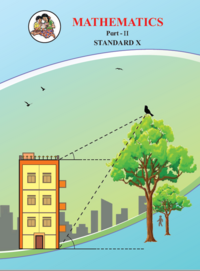Chapter 7: Mensuration

Chapter 7: Mensuration solutions [Pages 145 - 146]

Q 1 | Page 145

Find the volume of a cone if the radius of its base is 1.5 cm and its perpendicular height is 5 cm.

Q 2 | Page 145

Find the volume of a sphere of diameter 6 cm.

Q 3 | Page 145

Find the total surface area of a cylinder if the radius of its base is 5 cm and height is 40 cm.

Q 4 | Page 145

Find the surface area of a sphere of radius 7 cm.

Q 5 | Page 145

The dimensions of a cuboid are 44 cm, 21 cm, 12 cm. It is melted and a cone of height 24 cm is made. Find the radius of its base.

Q 6 | Page 145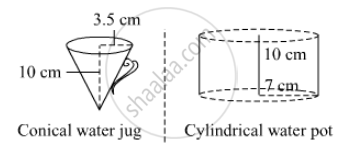Observe the measures of pots In the given figure. How many jugs of water can the cylindrical pot hold?

Q 7 | Page 145

A cylinder and a cone have equal bases. The height of the cylinder is 3 cm and the area of its base is 100 cm2 .The cone is placed
upon the cylinder. Volume of the solid figure so formed is 500 cm. Find the total height of the figure.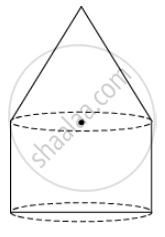Q 8 | Page 145

In the given figure, a toy made from a hemisphere, a cylinder and a cone is shown. Find the total area of the toy.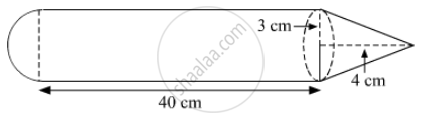Q 9 | Page 145

In the given figure, a cylindrical wrapper of flat tablets is shown. The radius of a tablet is 7 mm and its thickness is 5 mm. How many such tablets are wrapped in the wrapper?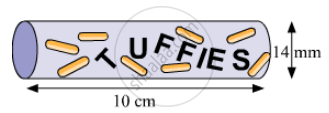Q 10 | Page 145

In the given figure shows a toy. Its lower part is a hemisphere and the upper part is a cone. Find the volume and surface area of the toy from the measures shown in the figure ($\pi = 3 . 14$)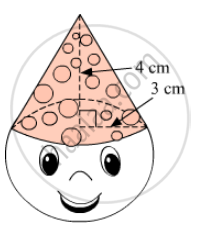Q 11 | Page 146

Find the surface area and the volume of a beach ball shown in the figure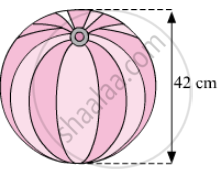Q 12 | Page 146

As shown in the figure, a cylindrical glass contains water. A metal sphere of diameter 2 cm is immersed in it. Find the volume of the water .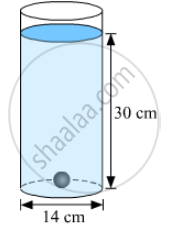Chapter 7: Mensuration solutions [Page 148]

Q 1 | Page 148
The radii of two circular ends of frustum shape bucket are 14 cm and 7 cm. Height of the bucket is 30 cm. How many liters of water it can hold ?
(1 litre = 1000 cm)
Q 2.1 | Page 148

The radii of ends of a frustum are 14 cm and 6 cm respectively and its height is 6 cm. Find its i) curved surface area

Q 2.2 | Page 148

The radii of ends of a frustum are 14 cm and 6 cm respectively and its height is 6 cm. Find its

ii) total surface area

Q 2.3 | Page 148

The radii of ends of a frustum are 14 cm and 6 cm respectively and its height is 6 cm. Find its

iii ) volume $\pi$ = 3.14)

Q 3 | Page 148

The circumferences of circular faces of a frustum are 132 cm and 88 cm and its height is 24 cm. To find the curved surface area of the frustum complete the following activity.( $\pi = \frac{22}{7}$)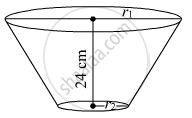circumference1 = 2

$\pi$ r1 = 132

r= $\frac{132}{\pi} = 134$
circumference2 =2
$\pi$ r2= 88
r2= $\frac{88}{2\pi} = 134$ slant height of frustum, l
$\sqrt{h^2 + \left( r_1 - r_2 \right)^2}$
$\sqrt{{1223}^2 + 1234 {}^2}\text{ textcolor} {\text{ white} }{}$
$= 1234 cm$
curved surface area of the frustum = $\pi$ (r+ r2 ) l
$\pi \times 123 \times 132$
$= 123 sq . cm .$

Chapter 7: Mensuration solutions [Pages 154 - 155]

Q 1 | Page 154

Radius of a circle is 10 cm. Measure of an arc of the crcleis 54°. Find the area of the sector associated with the arc. ($\pi$= 3.14 )

Q 2 | Page 154

Measure of an arc of a circle is 80 cm and its radius is 18 cm. Find the length of  the arc. ( $\pi$  = 3.14 )

Q 3 | Page 154

Radius of a sector of a circle is 3.5 cm and length of its arc is 2.2 cm. Find the area of the sector.

Q 4 | Page 154

Radius of a circle is 10 cm. Area of a sector of the sector is 100 cm2 . Find the area of its corresponding major sector. ( $\pi$  = 3.14 )

Q 5 | Page 154

Area of a sector of a circle of radius 15 cm is 30 cm2 . Find the length of the arc of the sector.

Q 6.1 | Page 154
In the given figure, radius of the circle is 7 cm and m ( arc MBN) = 60°,
find (1) Area of the circle .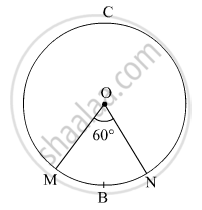Q 6.2 | Page 154
In the given figure, radius of the circle is 7 cm and m ( arc MBN) = 60°,
find   (2) A(O - MBN) .Q 6.3 | Page 154
In the given figure, radius of the circle is 7 cm and m ( arc MBN) = 60°,
find   (3) A(O - MCN) .Q 7 | Page 155

In the given figure, radius of circle is 3.4 cm and perimeter of sector P-ABC is 12.8 cm . Find A(P-ABC).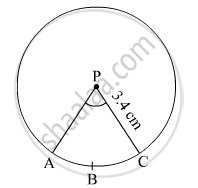Q 8 | Page 155

In the given figure, O is the centre of the sector. $\angle$ROQ =  $\angle$MON = 60° . OR = 7 cm, and   OM = 21 cm. Find the lengths of  arc RXQ and arc MYN. ( $\pi = \frac{22}{7}$)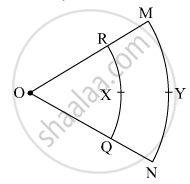Q 9 | Page 155

In the given figure, if A(P-ABC) = 154 cm2  radius of the circle is 14 cm, find

(1) ∠APC

(2) l ( arc ABC) .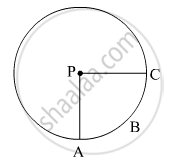Q 10.1 | Page 155
Radius of a sector of a circle is 7 cm. If measure of arc of the sector is -
(1) 30°  find the area of the sector
Q 10.2 | Page 155

Radius of a sector of a circle is 7 cm. If measure of arc of the sector is -

(2) 210° find the area of the sector

Q 10.3 | Page 155
Radius of a sector of a circle is 7 cm. If measure of arc of the sector is -
(3 ) three right angles; find the area of the sector
Q 11 | Page 155

The area of a minor sector of a circle is 3.85 cmand the measure of its central angle is 36°. Find the radius of the circle .

Q 12 | Page 155

In the given figure,

$\square$ PQRS is a rectangle. If PQ = 14 cm, QR = 21 cm, find the areas of the parts x, y and z .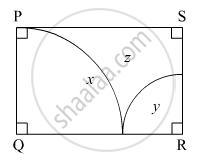Q 13.1 | Page 155
$∆$ LMN is an equilateral triangle. LM = 14 cm. As shown in figure, three sectors are drawn with vertices as centres and radius 7 cm.
Find,
(1) A ( $∆$ LMN)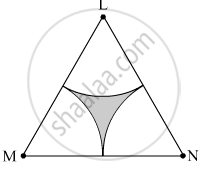Q 13.2 | Page 155
$∆$ LMN is an equilateral triangle. LM = 14 cm. As shown in figure, three sectors are drawn with vertices as centres and radius 7 cm.
Find,
(2) Area of any one of the sectors .Q 13.3 | Page 155
$∆$ LMN is an equilateral triangle. LM = 14 cm. As shown in figure, three sectors are drawn with vertices as centres and radius 7 cm.
Find,
(3) Total area of all the three sectors.Q 13.4 | Page 155
$∆$ LMN is an equilateral triangle. LM = 14 cm. As shown in figure, three sectors are drawn with vertices as centres and radius 7 cm.
Find,
(4) Area of the shaded region.Chapter 7: Mensuration solutions [Pages 159 - 160]

Q 1 | Page 159

In the given figure, A is the centre of the circle. $\angle$ABC = 45° and AC = 7 $\sqrt{2}$cm. Find the area of segment BXC.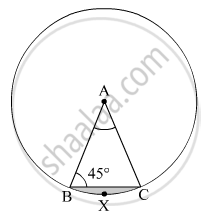Q 2 | Page 159

In the given figure, O is the centre of the circle. m ( arc PQR) = 60° OP = 10 cm. Find the area of the shaded region.( $\pi$= 3.14, $\sqrt{3}$= 1.73)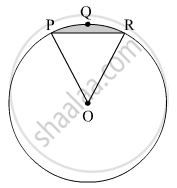Q 3 | Page 160

In the given figure, if A is the centre of the circle. $\angle$ PAR = 30°, AP = 7.5, find the area of the segment PQR ( $\pi$  = 3.14)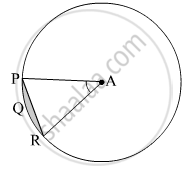Q 4 | Page 160

In the given figure, if O is the centre of the circle, PQ is a chord. $\angle$ POQ = 90°, area of shaded region is 114 cm2 , find the radius of the circle. $\pi$ = 3.14)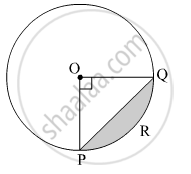Q 5 | Page 160

A chord PQ of a circle with radius 15 cm subtends an angle of 60° with the centre of the circle. Find the area of the minor as well as the major segment. ( $\pi$ = 3.14,  $\sqrt{3}$ = 1.73)

Chapter 7: Mensuration solutions [Pages 160 - 163]

Q 1.1 | Page 160
Choose the correct alternative answer for each of the following questions.
(1) The ratio of circumference and area of a circle is 2:7. Find its circumference.

• (A) $14\pi$

•   (B)   $\frac{7}{\pi}$

•  (C) 7$\pi$

•  (D)  $\frac{14}{\pi}$

Q 1.2 | Page 160
Choose the correct alternative answer for each of the following questions.
(2) If measure of an arc of a circle is 160° and its length is 44 cm, find the circumference of the circle.

• (A) 66 cm

• (B) 44 cm

•  (C) 160 cm

•  (D) 99 cm

Q 1.3 | Page 160

Choose the correct alternative answer for each of the following questions.

(3) Find the perimeter of a sector of a circle if its measure is 90° and radius is 7 cm .

• (A) 44 cm

•   (B) 25 cm

• (C) 36 cm

• (D) 56 cm

Q 1.4 | Page 160

Choose the correct alternative answer for each of the following questions.

(4) Find the curved surface area of a cone of radius 7 cm and height 24 cm.

• (A) 440 cm

• (B) 550 cm2

• (C) 330 cm

• (D) 110 cm2

Q 1.5 | Page 160

Choose the correct alternative answer for each of the following questions.

(5) The curved surface area of a cylinder is 440 cm 2 and its radius is 5 cm. Find its height.

• (A)  $\frac{44}{\pi}$ cm

•  (B) 22 $\pi$ CM

• (C) 44 $\pi$ CM

• (D) $\frac{22}{\pi}$ cm

Q 1.6 | Page 160

Choose the correct alternative answer for each of the following questions

(6) A cone was melted and cast into a cylinder of the same radius as that of the  base of the cone. If the height of the cylinder is 5 cm, find the height of the cone.

• (A) 15 cm

• (B) 10 cm

• (C) 18 cm

• (D) 5 cm

Q 1.7 | Page 160

Choose the correct alternative answer for each of the following questions

(7) Find the volume of a cube of side 0.01 cm.

• (A) 1 cm3

• B) 0.001 cm

• (C) 0.0001 cm3

•  (D) 0.000001 cm3

Q 1.8 | Page 160

Choose the correct alternative answer for each of the following questions

(8) Find the side of a cube of volume 1 m.

• (A) 1 cm

• (B) 10 cm

• (C) 100 cm

• (D)1000 cm

Q 2 | Page 161

A washing tub in the shape of a frustum of a cone has height 21 cm. The radii of the circular top and bottom are 20 cm and 15 cm respectively. What is the capacity of the tub ? ( $\pi = \frac{22}{7}$)

Q 3 | Page 161

Some plastic balls of radius 1 cm were melted and cast into a tube. The thickness, length and outer radius of the tube were 2 cm , 90 cm and 30 cm respectively. How many balls were melted to make the tube?

Q 4 | Page 161

A metal parallelopiped of measures 16 cm x 11 cm x 10 cm was melted to make coins. How many coins were made if the thickness and diameter of each coin was 2 mm and 2 cm respectively ?

Q 5 | Page 161

The diameter and length of a roller is 120 cm and 84 cm respectively. To level the ground, 200 rotations of the roller are required. Find the expenditure to level the ground at the rate of Rs. 10 per sq.m.

Q 6 | Page 161

The diameter and thickness of a hollow metals sphere are 12 cm and 0.01 m respectively. The density of the metal is 8.88 gm per cm. Find the outer surface area and mass of the sphere.

Q 7 | Page 161

A cylindrical bucket of diameter 28 cm and height 20 cm was full of sand. When the sand in the bucket was poured on the ground, the sand got converted into a shape of a cone. If the height of the cone was 14 cm, what was the base area of the cone ?

Q 8 | Page 161

The radius of a metallic sphere is 9 cm. It was melted to make a wire of diameter 4 mm. Find the length of the wire.

Q 9 | Page 161

The area of a sector of a circle of 6 cm radius is 15 $\pi$ sq.cm. Find the measure of the arc and length of the arc corresponding to the sector.

Q 10 | Page 161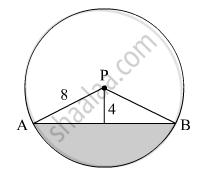In the given figure, seg AB is a chord of a circle with centre P. If PA = 8 cm and distance of chord AB from the centre P is 4 cm, find the area of the shaded portion. ( $\pi$ = 3.14, $\sqrt{3}$= 1.73 )

Q 11 | Page 162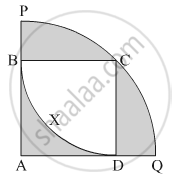In the given figure, square ABCD is inscribed in the sector A - PCQ. The radius of sector C - BXD is 20 cm. Complete the following activity to find the area of shaded region

Q 12 | Page 163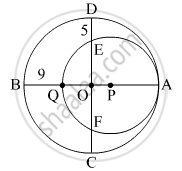In the given figure , two circles with centres O and P are touching internally  at point A. If BQ = 9, DE = 5, complete the following activity to find the radii of  the circles.

Chapter 7: Mensuration

Balbharati SSC Class 10 Mathematics 2Balbharati solutions for Class 10th Board Exam Geometry chapter 7 - Mensuration

Balbharati solutions for Class 10th Board Exam Geometry chapter 7 (Mensuration) include all questions with solution and detail explanation. This will clear students doubts about any question and improve application skills while preparing for board exams. The detailed, step-by-step solutions will help you understand the concepts better and clear your confusions, if any. Shaalaa.com has the Maharashtra State Board Textbook for SSC Class 10 Mathematics 2 solutions in a manner that help students grasp basic concepts better and faster.

Further, we at Shaalaa.com are providing such solutions so that students can prepare for written exams. Balbharati textbook solutions can be a core help for self-study and acts as a perfect self-help guidance for students.

Concepts covered in Class 10th Board Exam Geometry chapter 7 Mensuration are Conversion of Solid from One Shape to Another, Frustum of a Cone, Introduction of Surface Areas and Volumes, Length of an Arc, Problems Based on Areas and Perimeter Or Circumference of Circle, Sector and Segment of a Circle, Perimeter and Area of a Circle, Surface Area of a Combination of Solids, Euler's Formula, Areas of Sector and Segment of a Circle.

Using Balbharati Class 10th Board Exam solutions Mensuration exercise by students are an easy way to prepare for the exams, as they involve solutions arranged chapter-wise also page wise. The questions involved in Balbharati Solutions are important questions that can be asked in the final exam. Maximum students of Maharashtra State Board Class 10th Board Exam prefer Balbharati Textbook Solutions to score more in exam.

Get the free view of chapter 7 Mensuration Class 10th Board Exam extra questions for Geometry and can use Shaalaa.com to keep it handy for your exam preparation

S